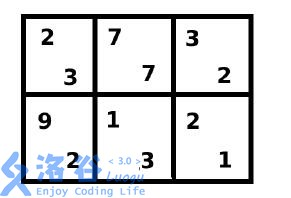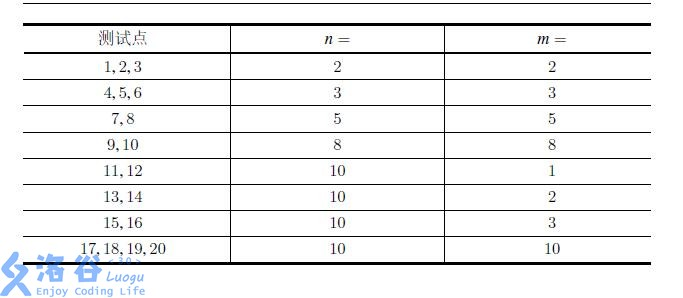# 洛谷4363 [九省联考2018]一双木棋chess

## 状压之后记忆化

Posted by yjjr's blog on April 10, 2018

# 题目

## 输入输出样例

### 输入样例#1

2 3
2 7 3
9 1 2
3 7 2
2 3 1


### 输出样例#1

2


## 说明• 菲菲下在第1 行第1 列（这是第一步时唯一可以落子的格子）；

• 牛牛下在第1 行第2 列；

• 菲菲下在第2 行第1 列；

• 牛牛下在第1 行第3 列；

• 菲菲下在第2 行第2 列；

• 牛牛下在第2 行第3 列（这是这一步时唯一可以落子的格子）；

• 填满棋盘，游戏结束，盘面如下。# 分析

. . . . .
. . . .
. . .
. .


# code

#include<iostream>
#include<cstdio>
#include<cstdlib>
#include<cmath>
#include<cstring>
#include<algorithm>
#include<map>
#define rep(i,a,b) for(int i=a;i<=b;i++)
#define dep(i,a,b) for(int i=a;i>=b;i--)
#define ll long long
#define mem(x,num) memset(x,num,sizeof x)
#define reg(x) for(int i=last[x];i;i=e[i].next)
using namespace std;
inline ll read(){
ll f=1,x=0;char ch=getchar();
while(ch<'0'||ch>'9'){if(ch=='-')f=-1;ch=getchar();}
while(ch>='0'&&ch<='9'){x=x*10+ch-'0';ch=getchar();}
return x*f;
}
//**********head by yjjr**********
#define inf 1e9
const int maxn=26;
ll End;int n,m,num[maxn],a[maxn][maxn],b[maxn][maxn];
map<ll,int> mp;
inline int unzip(ll sta){
int s=0;
dep(i,n,1)s+=(num[i]=(sta%(m+1))),sta/=(m+1);
return s&1;
}//判断当前该谁下棋
inline ll zip(){
ll s=0;
rep(i,1,n)s=s*(m+1)+num[i];
return s;
}//压缩状态
inline int dfs(ll sta){
if(mp.find(sta)!=mp.end())return mp[sta];
if(sta==End)return 0;//如果搜索到最终状态，那么就退出
int opt=unzip(sta),ans=opt?inf:-inf;
if(num<m){
++num;
if(opt)ans=min(ans,dfs(zip())-b[num]);
else ans=max(ans,dfs(zip())+a[num]);
num--;
}
rep(i,2,n)
if(num[i-1]>num[i]){
++num[i];
if(opt)ans=min(ans,dfs(zip())-b[i][num[i]]);
else ans=max(ans,dfs(zip())+a[i][num[i]]);
num[i]--;
}
return mp[sta]=ans;
}
int main(){
n=read(),m=read();
rep(i,1,n)rep(j,1,m)a[i][j]=read();
rep(i,1,n)rep(j,1,m)b[i][j]=read();
rep(i,1,n)num[i]=m;
End=zip();
dfs(0);
cout<<mp<<endl;
return 0;
}